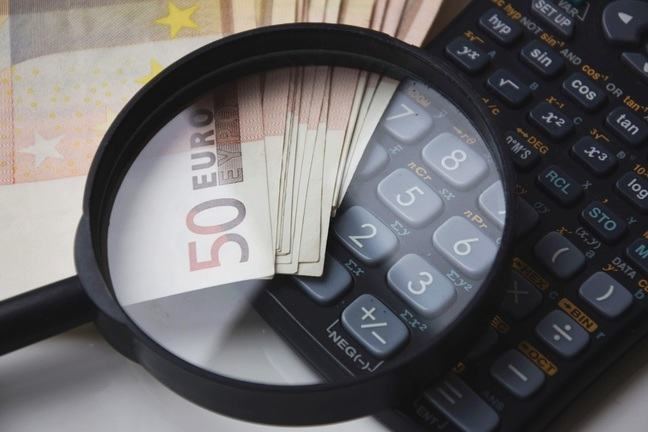## Want to keep learning?

This content is taken from the SOAS University of London's online course, Risk Management in the Global Economy. Join the course to learn more.
2.13

## SOAS University of London# How does diversification work?

In the previous step we talked about ice-cream, raincoats and umbrellas, correlation, and the potential for diversification. Sometimes it is also useful to be able to present these relationships mathematically. The maths helps because it allows us to express the ideas very precisely.

In this step we will focus on how to use diversification to reduce the risk of a portfolio. It will give you a clearer picture of how you can reduce your risk, by investing in assets whose returns on average are not perfectly related.

The step proceeds as follows. We consider two assets that have the same expected return and the same standard deviation. If we formed a portfolio consisting of only one asset (or the other asset) the risk of the portfolio would be the same as the risk of the asset. Then we ask: is it possible to form a portfolio combining the two assets, such that the risk of the portfolio is less than the risk of holding one or other of the assets separately?

Let us consider two assets, $$AB$$ and $$XY$$, with the same expected return, 5%:

${{{\mu}_A}_B}=E({{{R}_A}_B})=0.05=5\%$ ${{{\mu}_X}_Y}=E({{{R}_X}_Y})=0.05=5\%$

and with the same risk measured by their standard deviation, 4%:

${{{\sigma}_A}_B}=0.04=4\%$ ${{{\sigma}_X}_Y}=0.04=4\%$

We now form a portfolio, $$P$$, where assets $$AB$$ and $$XY$$ are held in equal proportion. You saw in step 2.3 that the return on the portfolio will be an average of the returns on each one of the assets:

$R_P={1 \over 2}{{{R}_A}_B}+{1 \over 2}{{{R}_X}_Y}$

The expected return on $$P$$ is:

$\mu_P=E(R_P)={1 \over 2}{{{\mu}_A}_B}+{1 \over 2}{{{\mu}_X}_Y}+{1 \over 2}{0.05}+{1 \over 2}{0.05}=0.05=5\%$

Therefore, the expected return on the portfolio will be the same as the expected return on each one of the assets.

But what about the variability of the returns on the portfolio? The variance of the portfolio return depends on the variance of the returns on the two assets, but also on how the returns on the two assets vary together, that is, on their covariance. When the two assets are held in equal proportions, the variance of the portfolio is:

${{\sigma}^2_P}=\left({1 \over 2}\right)^2\, {{{\sigma}^2_A}_B}+\left({1 \over 2}\right)^2 \,{{{\sigma}^2_X}_Y}+2\left({1 \over 2}\right)\left({1 \over 2}\right) \,{{{\sigma}_A}_B},{_X}{_Y}$

where $${{{\sigma}_A}_B},{_X}{_Y}$$ is the covariance between the assets.

Covariance can range potentially from minus infinity to plus infinity, which is hard to think about. Therefore it is more helpful if we express the covariance between $$AB$$ and $$XY$$ as the product of the correlation coefficients between the two assets, $${{{\rho}_A}_B},{_X}{_Y}$$, and of their standard deviations, $${{{\sigma}_A}_B}$$ and $${{{\sigma}_X}_Y}$$:

${{{\sigma}_A}_B},{_X}{_Y}={{{\rho}_A}_B},{_X}{_Y} \times {{{\sigma}_A}_B} \times {{{\sigma}_X}_Y}$

The correlation coefficient is a measure of how the returns on the assets vary together, and can take values between -1 and 1. It is positive when the covariance is positive, and negative when the covariance is negative.

The variance of the portfolio, $${{\sigma}^2_P}$$, is thus given by:

${{\sigma}^2_P}=\left({1 \over 4}\right){(0.04)^2}+\left({1 \over 4}\right){(0.04)^2}+2\left({1 \over 2}\right)\left({1 \over 2}\right) \times {{{\rho}_A}_B},{_X}{_Y} \times 0.04 \times 0.04$

If we carry out all the calculations and simplify, the above formula becomes:

${{\sigma}^2_P}=0.0008 \times (1+{{{\rho}_A}_B},{_X}{_Y})$

This equation emphasises the importance of the correlation coefficient.

The following table presents values for the correlation coefficient, in a range going from +1 to -1, and the corresponding values for the variance and the standard deviation of the portfolio return.

What happens to the standard deviation of the portfolio return, shown in the right-hand column, as we move down the table?

$${{{\rho}_A}_B},{_X}{_Y}$$ $${{\sigma}^2_P}$$ $${{\sigma}_P}$$
1 0.0016 0.040
0.5 0.0012 0.035
0 0.0008 0.028
- 0.5 0.0004 0.020
- 1 0 0.000

When the correlation coefficient is 1, there is no gain from diversification: the standard deviation of the portfolio is 0.04 = 4%, which is the same as the standard deviation of the individual assets.

But look at the other rows. The standard deviation of the portfolio is lower than that of the assets considered separately. When the two assets are less than perfectly positively correlated, diversification can be effective in reducing the risk of the portfolio.

In the bottom row the returns on the two assets are perfectly negatively correlated. When the correlation coefficient is equal to -1, the portfolio standard deviation becomes zero: we have completely eliminated uncertainty, and the portfolio is now riskless.

Think about that result. Are you convinced by it? If it helps, think back to the umbrella firm and the ice-cream firm. What does it mean if the returns on their shares are perfectly negatively correlated?